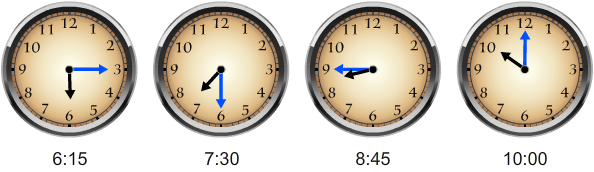# Patterns with Time

Print Rate 0 stars Common Core
Lesson size:
Message preview:
Someone you know has shared lesson with you:

To play this lesson, click on the link below:

https://www.turtlediary.com/lesson/patterns-with-time.html

Hope you have a good experience with this site and recommend to your friends too.

Login to rate activities and track progress.
Login to rate activities and track progress.

Sometimes you will be given a pattern of time, either written or in pictures. In such cases, you have to examine them and figure out what the pattern is.

Here are the steps you need to follow:

• Determine how much time has been added or subtracted between the first two times.
• Double check to make sure the same amount of time passes between the 2nd and 3rd time. If it does, you have found the pattern.
• Add or subtract the same amount of time to continue the pattern.

Let's take a look at some examples:

## Example 1

The deliveries occur at these times: 4:10 PM, 4:30 PM, 4:50 PM. If this pattern continues, when will the next delivery occur?

## Solution :

First we need to see how much time has passed between the first two deliveries:

Let's subtract the minutes:

30 - 10 = 20, so 20 minutes have passed.

We now need to see if this pattern stays true.

Did 20 minutes pass between 4:30 and 4:50?

50 - 30 = 20,

Yes, the pattern is 20 minutes.

We must find the next time by adding 20 minutes to 4:50 PM, but we can't just add 20 to 50 because we'd get 4:70 and that time doesn't exist.

Let's start by thinking how much time will pass until 5:00. It would take 10 minutes (because there are 60 minutes in an hour and it's already 4:50).

That leaves how much more time?

20 minutes - 10 minutes = 10 minutes.

What is 10 minutes past 5:00?

It is 5:10 PM

Yes, it makes sense that 5:10 PM is 20 minutes after 4:50 PM.

## Example 2

Determine what time will come next in the pattern given below:## Solution :

First we need to tell the time on each clock:

6:15, 7:30, 8:45, 10:00

Now we need to determine how much time passed in between the first two clocks.

1 hour past 6:15 is 7:15

How many minutes are there between 7:15 and 7:30?

It is 15 because 30 - 15 = 15.

So, 1 hour and 15 minutes have passed.

Let's make sure this is the right pattern by checking the next set.

Is there 1 hour and 15 minutes in between 7:30 and 8:45?

Yes, so this is the right pattern.

We could check if the pattern is true for 8:45 and 10:00 (which it is), but we don't have to because if it's true for two sets, it will continue to be true for the rest of the pattern.

We now need to find the next time by adding 1 hour 15 minutes to 10:00.

1 hour past 10:00 is 11:00 and then 15 minutes later is 11:15.

(We don't know whether or not it is AM or PM)

Does the answer make sense? Yes, it does because 11:15 is 1 hour 15 minutes past 10:00.

## Example 3

Eric is timing his older brother as he runs around the track. The times after his first three laps were 4:10 PM, 4:12 PM, and 4:14 PM. If he can keep up this pace and the pattern continues, what time will it be after he finishes 8 laps in total?

## Solution :

Let's start by finding the pattern.

There are 2 minutes in between 4:10 and 4:12,

There are 2 minutes in between 4:12 and 4:14,

So we know this is our pattern.

We can easily continue this pattern, but we have to find out what will be the time after 8 laps.

So let's expand this pattern to 8 laps:

4:10, 4:12, 4:14, 4:16, 4:18, 4:20, 4:22, 4:24

Answer: After 8 laps the time will be 4:24 PM.

Does this answer make sense? Yes, this is a reasonable time for how fast he is running.

## Patterns with Time

Determining patterns with time:

• Determine how much time has been added or subtracted between the first two times.
• Double check to make sure the same amount of time passes between the 2nd and 3rd time. If it does, you have found the pattern.
• Add or subtract the same amount of time to continue the pattern.

## Similar Lessons

Become premium member to get unlimited access.